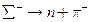Chapter 33, Problem 31PE

Chapter
Section
Textbook Problem

(a) Is the decaypossible considering the appropriate conservation laws? Stale why or why not. (b) Write the decay in terms of the quark constituents of the particles.

To determine

(a)

Whether the decay Σn+π is possible as per the conservation laws.

Explanation

Given info:

Given reaction is Σn+π

Calculation:

We can verify whether the decay Σn+π is possible by checking the following

conservation laws.

Decay:

Σn+π

Charge:1  0  1  conserved

B:  +1  +1  0

To determine

(b)

The equation Σn+π in terms of quark structure.

Still sussing out bartleby?

Check out a sample textbook solution.

See a sample solution

The Solution to Your Study Problems

Bartleby provides explanations to thousands of textbook problems written by our experts, many with advanced degrees!

Get Started